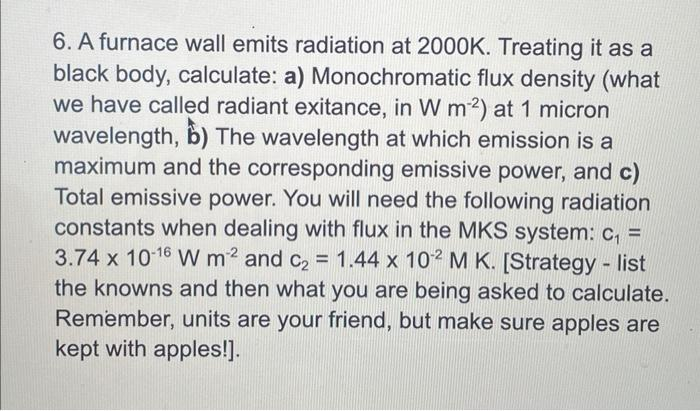# (Solved): 6. A furnace wall emits radiation at $$2000 \mathrm{~K}$$. Treating it as a black body, calculate ...6. A furnace wall emits radiation at $$2000 \mathrm{~K}$$. Treating it as a black body, calculate: a) Monochromatic flux density (what we have called radiant exitance, in $$\mathrm{W} \mathrm{m}^{-2}$$ ) at 1 micron wavelength, b) The wavelength at which emission is a maximum and the corresponding emissive power, and c) Total emissive power. You will need the following radiation constants when dealing with flux in the MKS system: $$c_{1}=$$ $$3.74 \times 10^{-16} \mathrm{~W} \mathrm{~m}^{-2}$$ and $$\mathrm{c}_{2}=1.44 \times 10^{-2} \mathrm{M} \mathrm{K}$$. [Strategy - list the knowns and then what you are being asked to calculate. Remember, units are your friend, but make sure apples are kept with apples!].

We have an Answer from Expert